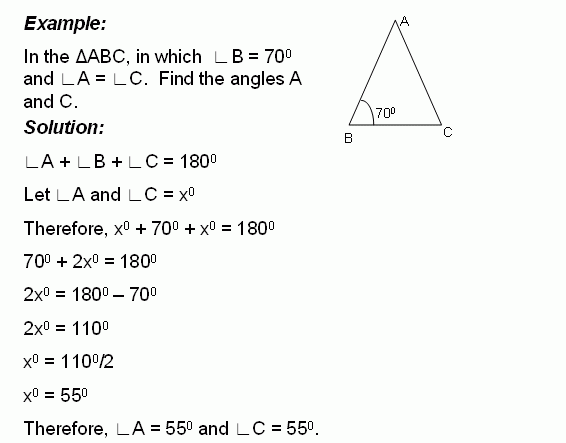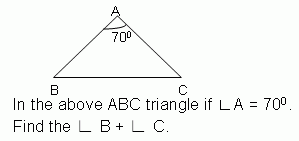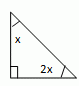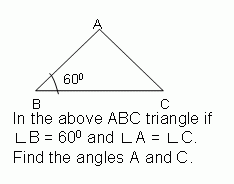Email us to get an instant 20% discount on highly effective K-12 Math & English kwizNET Programs!

#### Online Quiz (WorksheetABCD)

Questions Per Quiz = 2 4 6 8 10

### Geometry2.7 Find the Angles of TriangleDirections: Solve the following problems. Also write at least 10 examples of your own.
 Q 1:100 degrees105 degrees110 degrees Q 2: Find the angles of the triangle.30, 60, 90 triangle60, 60, 60 triangle45, 45, 90 triangle Q 3:45 degrees60 degrees55 degrees Q 4: Find the angles of the triangle and classify based on the angles.20, 60, 10030, 60, 9045, 45, 90 Q 5:90 degrees100 degrees95 degrees Q 6: Find the angles of the triangle and classify based on the angles.26, 64, right angle triangle32, 96, right angle triangle30, 60, right angle triangle Q 7: The measure of angle x is _____ degrees.Answer: Q 8:60 degrees75 degrees65 degrees Question 9: This question is available to subscribers only! Question 10: This question is available to subscribers only!